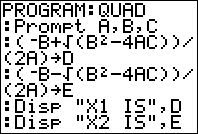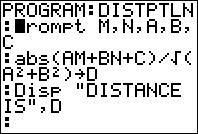## Wednesday, January 18, 2012

### Some handy programs for your TI calculator

 These programs might come in handy, and they are all based on the same 3 programming steps: Get the calculator to prompt you for some variable(s), (PROMPT) Tell the calculator what calculations to do to those variable(s), and where to store the result, (STORE) Tell the calculator to tell you the result. (DISPLAY) Here are my programs for distance between 2 points, solutions to a quadratic equation, and distance between a point and a line:Distance between points (A, B) and (C, D)Solutions to quadratic equation ax² + bx + c = 0Distance between line Ax + By + C = 0 and point (M, N) If you have written any programs, don't hesitate to share them on your blog!